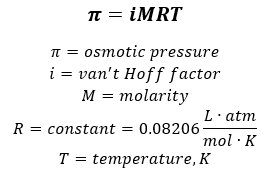# Problem: Using the van't Hoff factors in the table below, calculate the mass of solute required to make each aqueous solution.Van't Hoff factors at 0.05 m concentration in aqueous solutionSoluteExpectedMeasuredNonelectrolyte11NaCl21.9MgSO421.3MgCl232.7K2SO432.6FeCl343.4Calculate the mass of solute required to make 261 mL of a magnesium sulfate solution that has an osmotic pressure of 3.91 atm at 307 K .

###### FREE Expert Solution

Recall the equation used to calculate for osmotic pressure:Calculate the Molarity of the solution:

88% (106 ratings)###### Problem Details
Using the van't Hoff factors in the table below, calculate the mass of solute required to make each aqueous solution.
Van't Hoff factors at 0.05 m concentration in aqueous solution
 Solute Expected Measured Nonelectrolyte 1 1 NaCl 2 1.9 MgSO4 2 1.3 MgCl2 3 2.7 K2SO4 3 2.6 FeCl3 4 3.4

Calculate the mass of solute required to make 261 mL of a magnesium sulfate solution that has an osmotic pressure of 3.91 atm at 307 K .

Frequently Asked Questions

What scientific concept do you need to know in order to solve this problem?

Our tutors have indicated that to solve this problem you will need to apply the The Colligative Properties concept. You can view video lessons to learn The Colligative Properties. Or if you need more The Colligative Properties practice, you can also practice The Colligative Properties practice problems.

What professor is this problem relevant for?

Based on our data, we think this problem is relevant for Professor Kobko-Litskevitch's class at HUNTER.